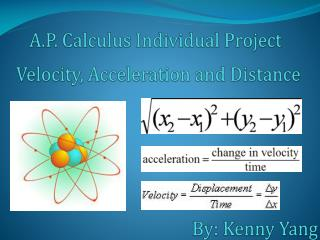# Velocity, Acceleration and Distance - PowerPoint PPT PresentationDownload PresentationVelocity, Acceleration and Distance

Velocity, Acceleration and DistanceDownload Presentation## Velocity, Acceleration and Distance

- - - - - - - - - - - - - - - - - - - - - - - - - - - E N D - - - - - - - - - - - - - - - - - - - - - - - - - - -
##### Presentation Transcript

1. A.P. Calculus Individual Project Velocity, Acceleration andDistance By:Kenny Yang

2. A particle moves along the x -axis so that its velocity at time t is given by At time t = 0, the particle is at position x = 1(a) Find the acceleration of the particle at time t = 2. Is the speed of the particle increasing at t = 2? Why or why not?(b) Find all times t in the open interval 0 < t < 3, when the particle changes direction. Justify your answer.(c) Find the total distance traveled by the particle from t = 0 until time t = 3.(d) During the time interval0 t 3, what is the greatest distance between the particle and the origin? Show the work that leads to your answer.

3. (a) Find the acceleration of the particle at time t = 2. Is the speed of the particle increasing at t = 2? Why or why not?

4. (b) Find all times t in the open interval 0 < t < 3, when the particle changes direction. Justify your answer.

5. (c) Find the total distance traveled by the particle from t = 0 until time t = 3.

6. (d) During the time interval0 t 3, what is the greatest distance between the particle and the origin? Show the work that leads to your answer.

7. Work Cited "AP Calculus AB 2003 Scoring Guidelines." Apcentral.collegeboard.com. College Entrance Examination Board. Web. 26 May 2011. <http://apcentral.collegeboard.com/apc/members/repository/ap03_sg_calculus_ab_26472.pdf>.

8. The End